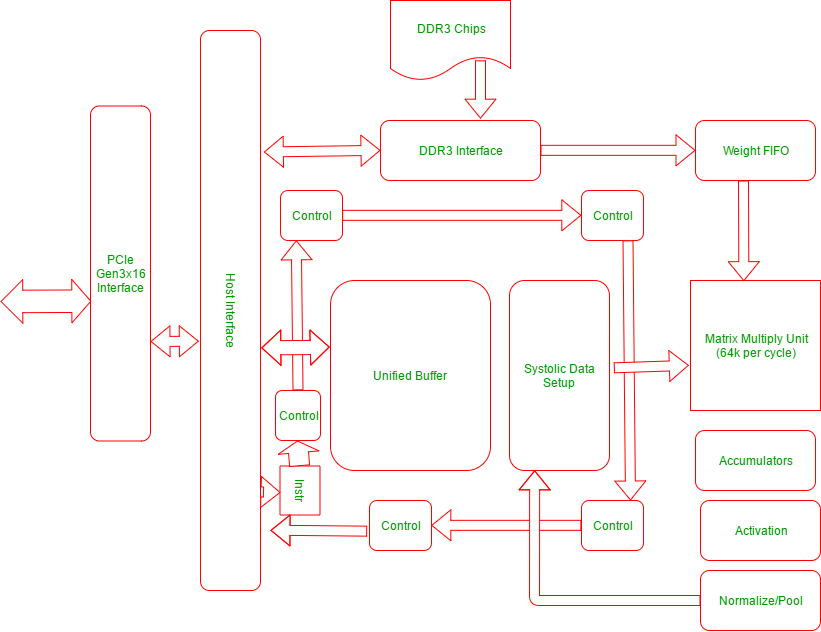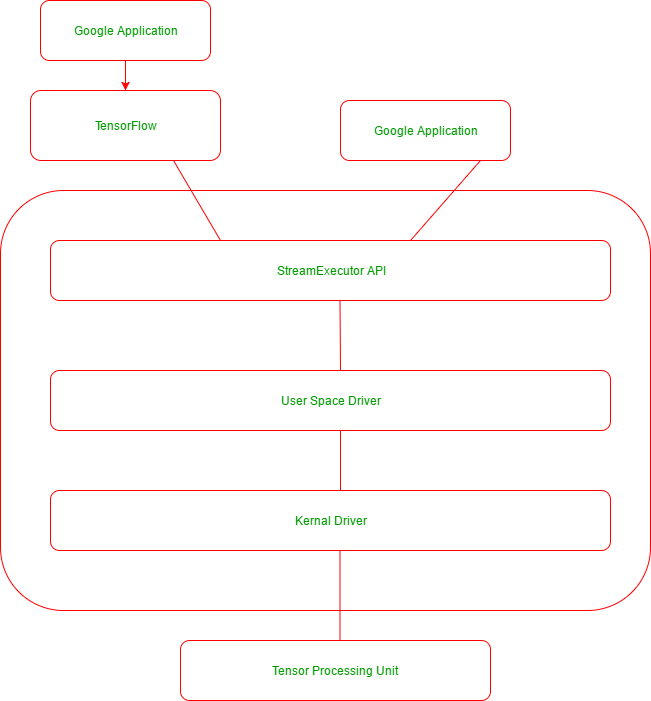Skip to content
Related Articles
Understanding Tensor Processing Units
• Last Updated : 04 Sep, 2018

What is a Tensor Processing Unit?

With machine learning gaining its relevance and importance everyday, the conventional microprocessors have proven to be unable to effectively handle it, be it training or neural network processing. GPUs, with their highly parallel architecture designed for fast graphic processing proved to be way more useful than CPUs for the purpose, but were somewhat lacking. Therefore, in order to combat this situation, Google developed an AI accelerator integrated circuit which would be used by its TensorFlow AI framework. This device has been named TPU (Tensor Processing Unit). The chip has been designed for Tensorflow Framework.

What is TensorFlow Framework?

TensorFlow is an open source library developed by Google for its internal use. Its main usage is in machine learning and dataflow programming. TensorFlow computations are expressed as stateful dataflow graphs. The name TensorFlow derives from the operations that such neural networks perform on multidimensional data arrays. These arrays are referred to as “tensors”. TensorFlow is available for Linux distributions, Windows, and MacOS.

TPU Architecture

The following diagram explains the physical architecture of the units in a TPU:The TPU includes the following computational resources:

• Matrix Multiplier Unit (MXU): 65, 536 8-bit multiply-and-add units for matrix operations.
• Unified Buffer (UB): 24MB of SRAM that work as registers
• Activation Unit (AU): Hardwired activation functions.

There are 5 major high level instruction sets devised to control how the above resources work. They are as follows:

TPU InstructionFunction
Read_Host_MemoryRead data from memory
Read_WeightsRead weights from memory
MatrixMultiply/ConvolveMultiply or convolve with the data and weights, accumulate the results
ActivateApply activation functions
Write_Host_MemoryWrite result to memory

The following is the diagram the application stack maintained by the google applications that use TensorFlow and TPU:Advantages of TPU

The following are some notable advantages of TPUs:

1. Accelerates the performance of linear algebra computation, which is used heavily in machine learning applications.
2. Minimizes the time-to-accuracy when you train large, complex neural network models.
3. Models that previously took weeks to train on other hardware platforms can converge in hours on TPUs.

When to use a TPU

The following are the cases where TPUs are best suited in machine learning:

• Models dominated by matrix computations.
• Models with no custom TensorFlow operations inside the main training loop.
• Models that train for weeks or months
• Larger and very large models with very large effective batch sizes.My Personal Notes arrow_drop_up